HomeForum of Mathematics, Sigma

#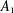$A_{1}$ -TYPE SUBGROUPS CONTAINING REGULAR UNIPOTENT ELEMENTS

Published online by Cambridge University Press:  24 April 2019

Corresponding

## Abstract

Let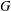$G$ be a simple exceptional algebraic group of adjoint type over an algebraically closed field of characteristic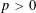$p>0$ and let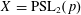$X=\text{PSL}_{2}(p)$ be a subgroup of$G$ containing a regular unipotent element$x$ of$G$ . By a theorem of Testerman,$x$ is contained in a connected subgroup of$G$ of type$A_{1}$ . In this paper we prove that with two exceptions,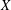$X$ itself is contained in such a subgroup (the exceptions arise when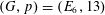$(G,p)=(E_{6},13)$ or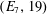$(E_{7},19)$ ). This extends earlier work of Seitz and Testerman, who established the containment under some additional conditions on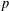$p$ and the embedding of$X$ in$G$ . We discuss applications of our main result to the study of the subgroup structure of finite groups of Lie type.

## MSC classification

Type
Research Article
Information
Creative Commons
This is an Open Access article, distributed under the terms of the Creative Commons Attribution licence (http://creativecommons.org/licenses/by/4.0/), which permits unrestricted re-use, distribution, and reproduction in any medium, provided the original work is properly cited.

## References

Alperin, J. L., Local Representation Theory, Cambridge Studies in Advanced Mathematics, 11 (Cambridge University Press, Cambridge, 1986).CrossRefGoogle Scholar
Borel, A. and Tits, J., ‘Éléments unipotents et sous-groupes paraboliques de groupes réductifs. I’, Invent. Math. 12 (1971), 95104.CrossRefGoogle Scholar
Bosma, W., Cannon, J. and Playoust, C., ‘The Magma algebra system I: the user language’, J. Symbolic Comput. 24 (1997), 235265.CrossRefGoogle Scholar
Bourbaki, N., Groupes et algebrès de Lie (Chapitres 4, 5 et 6) (Hermann, Paris, 1968).Google Scholar
Carter, R. W., Simple Groups of Lie Type (Wiley, London, 1972).Google Scholar
Carter, R. W., Finite Groups of Lie Type: Conjugacy Classes and Complex Characters (Wiley, London, 1985).Google Scholar
Cohen, A. M. and Griess, R. L., ‘On finite subgroups of the complex Lie group of type E 8 ’, Proc. Sympos. Pure Math. 47 (1987), 367405.CrossRefGoogle Scholar
Cooperstein, B. N., ‘Maximal subgroups of G 2(2 n )’, J. Algebra 70 (1981), 2336.CrossRefGoogle Scholar
Craven, D. A., ‘Maximal PSL2 subgroups of exceptional groups of Lie type’, Mem. Amer. Math. Soc. to appear.Google Scholar
Gorenstein, D., Lyons, R. and Solomon, R., The Classification of the Finite Simple Groups, Number 3, Mathematical Surveys and Monographs, 40 (Amererican Mathematical Society, Providence, RI, 1998).Google Scholar
Guralnick, R. and Malle, G., ‘Rational rigidity for E 8(p)’, Compos. Math. 150 (2014), 16791702.CrossRefGoogle Scholar
Janusz, G. J., ‘Indecomposable representations of groups with a cyclic Sylow subgroup’, Trans. Amer. Math. Soc. 125 (1966), 288295.CrossRefGoogle Scholar
Kleidman, P. B., ‘The maximal subgroups of the Chevalley groups G 2(q) with q odd, the Ree groups 2 G 2(q), and their automorphism groups’, J. Algebra 117 (1988), 3071.CrossRefGoogle Scholar
Lawther, R., ‘Jordan block sizes of unipotent elements in exceptional algebraic groups’, Comm. Algebra 23 (1995), 41254156.CrossRefGoogle Scholar
Lawther, R. and Testerman, D. M., ‘ A 1 subgroups of exceptional algebraic groups’, Mem. Amer. Math. Soc. 674 (1999), viii+131 pp.Google Scholar
Liebeck, M. W., Saxl, J. and Testerman, D. M., ‘Simple subgroups of large rank in groups of Lie type’, Proc. Lond. Math. Soc. 72 (1996), 425457.CrossRefGoogle Scholar
Liebeck, M. W. and Seitz, G. M., ‘Subgroups generated by root elements in groups of Lie type’, Ann. Math. 139 (1994), 293361.CrossRefGoogle Scholar
Liebeck, M. W. and Seitz, G. M., ‘On the subgroup structure of exceptional groups of Lie type’, Trans. Amer. Math. Soc. 350 (1998), 34093482.CrossRefGoogle Scholar
Liebeck, M. W. and Seitz, G. M., ‘The maximal subgroups of positive dimension in exceptional algebraic groups’, Mem. Amer. Math. Soc. 802 (2004), vi+227 pp.Google Scholar
Liebeck, M. W. and Testerman, D. M., ‘Irreducible subgroups of algebraic groups’, Q. J. Math. 55 (2004), 4755.CrossRefGoogle Scholar
Litterick, A. J., ‘Finite simple subgroups of exceptional algebraic groups’, PhD Thesis, Imperial College London, 2013.Google Scholar
Litterick, A. J., ‘On non-generic finite subgroups of exceptional algebraic groups’, Mem. Amer. Math. Soc. 1207 (2018), v+156 pp.Google Scholar
Malle, G., ‘The maximal subgroups of  2 F 4(q 2)’, J. Algebra 139 (1991), 5269.CrossRefGoogle Scholar
Malle, G. and Testerman, D., Linear Algebraic Groups and Finite Groups of Lie Type, Cambridge Advanced Studies in Mathematics, 133 (Cambridge University Press, Cambridge, 2011).CrossRefGoogle Scholar
Moody, R. V. and Patera, J., ‘Characters of elements of finite order in Lie groups’, SIAM J. Algebr. Discrete Methods 5 (1984), 359383.CrossRefGoogle Scholar
Proud, R., Saxl, J. and Testerman, D. M., ‘Subgroups of type A 1 containing a fixed unipotent element in an algebraic group’, J. Algebra 231 (2000), 5366.CrossRefGoogle Scholar
Saxl, J. and Seitz, G. M., ‘Subgroups of algebraic groups containing regular unipotent elements’, J. Lond. Math. Soc. 55 (1997), 370386.CrossRefGoogle Scholar
Seitz, G. M., ‘Unipotent elements, tilting modules, and saturation’, Invent. Math. 141 (2000), 467502.CrossRefGoogle Scholar
Seitz, G. M. and Testerman, D. M., ‘Extending morphisms from finite to algebraic groups’, J. Algebra 131 (1990), 559574.CrossRefGoogle Scholar
Seitz, G. M. and Testerman, D. M., ‘Subgroups of type A 1 containing semiregular unipotent elements’, J. Algebra 196 (1997), 595619.CrossRefGoogle Scholar
Serre, J.-P., ‘Exemples de plongements des groupes PSL2(F p ) dans des groupes de Lie simples’, Invent. Math. 124 (1996), 525562.CrossRefGoogle Scholar
Steinberg, R., ‘Representations of algebraic groups’, Nagoya Math. J. 22 (1963), 3356.CrossRefGoogle Scholar
Steinberg, R., ‘Endomorphisms of linear algebraic groups’, Mem. Amer. Math. Soc. 80 (1968), 108 pp.Google Scholar
Testerman, D. M., ‘The construction of the maximal A 1 ’s in the exceptional algebraic groups’, Proc. Amer. Math. Soc. 116 (1992), 635644.Google Scholar
Testerman, D. M., ‘ A 1 -type overgroups of elements of order p in semisimple algebraic groups and the associated finite groups’, J. Algebra 177 (1995), 3476.CrossRefGoogle Scholar
Testerman, D. M. and Zalesski, A., ‘Irreducibility in algebraic groups and regular unipotent elements’, Proc. Amer. Math. Soc. 141 (2013), 1328.CrossRefGoogle Scholar
Weisfeiler, B., ‘On one class of unipotent subgroups of semisimple algebraic groups’, Preprint, 2000, arXiv:0005149.Google Scholar

### Full text views

Full text views reflects PDF downloads, PDFs sent to Google Drive, Dropbox and Kindle and HTML full text views.

Total number of HTML views: 0
Total number of PDF views: 213 *
View data table for this chart

* Views captured on Cambridge Core between 24th April 2019 - 18th January 2021. This data will be updated every 24 hours.

Access
Open access
Hostname: page-component-77fc7d77f9-fgqm6 Total loading time: 0.263 Render date: 2021-01-18T05:13:21.954Z Query parameters: { "hasAccess": "1", "openAccess": "1", "isLogged": "0", "lang": "en" } Feature Flags last update: Mon Jan 18 2021 04:56:00 GMT+0000 (Coordinated Universal Time) Feature Flags: { "metrics": true, "metricsAbstractViews": false, "peerReview": true, "crossMark": true, "comments": true, "relatedCommentaries": true, "subject": true, "clr": true, "languageSwitch": true, "figures": false, "newCiteModal": false, "shouldUseShareProductTool": true, "shouldUseHypothesis": true, "isUnsiloEnabled": true }

# Send article to Kindle

Note you can select to send to either the @free.kindle.com or @kindle.com variations. ‘@free.kindle.com’ emails are free but can only be sent to your device when it is connected to wi-fi. ‘@kindle.com’ emails can be delivered even when you are not connected to wi-fi, but note that service fees apply.

Find out more about the Kindle Personal Document Service.$A_{1}$-TYPE SUBGROUPS CONTAINING REGULAR UNIPOTENT ELEMENTS Available formats × # Send article to Dropbox To send this article to your Dropbox account, please select one or more formats and confirm that you agree to abide by our usage policies. If this is the first time you use this feature, you will be asked to authorise Cambridge Core to connect with your <service> account. Find out more about sending content to Dropbox.$A_{1}$ -TYPE SUBGROUPS CONTAINING REGULAR UNIPOTENT ELEMENTS
Available formats
×

# Send article to Google Drive

To send this article to your Google Drive account, please select one or more formats and confirm that you agree to abide by our usage policies. If this is the first time you use this feature, you will be asked to authorise Cambridge Core to connect with your <service> account. Find out more about sending content to Google Drive.$A_{1}\$ -TYPE SUBGROUPS CONTAINING REGULAR UNIPOTENT ELEMENTS
Available formats
×
×